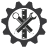SuperTeacherTools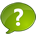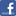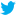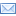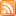4th Grade Math Vocabulary Speed Match Review Game

This game has been played times
The best time ever was at seconds.
The best time today is at seconds.
How To Play:
Drag the squares in the gray area and drop them in the matching squares in the blue area below.
If you drop a square in the correct spot, it will disappear.
If you make a mistake, the square will return to its original spot.
Good luck!

Let's Play Speed Match!

A point
The range
A vertex (vertices)
A sum
A prime number
A product is the answer to multiplication. A quotient is the answer to a division problem.
The commutative property or the order property
Inverse operations
A value
Parenthesis
Stem is the tens digit, Leaf is the ones digit
The mean or average
A remainder
A ray
An expression
A period
Order of operations
The mode
The difference
Perpendicular lines
A variable
A line
An equation
The median
A tally mark
What is the number of items that occurs the most often in a set of data?
What is a straight path in a plane, extending in both directions with no endpoints?
What is the tens digit in a stem and leaf plot? What is the ones digit?
What is a number sentence which shows that 2 quantities are equal?
What is a group of three digits separated by commas in a multi-digit number?
What are operations that undo each other, opposite operations?
What is a whole number great than 1 that has only two factors, 1 and itself?
What is the answer to an addition problem?
What is a line that represents one occurrence, when you mark your fifth occurrence it is marked with a diagonal line?
What is a point at which two rays or line segments meet?
What is the order in which you should figure your steps in a multi-operational problem?
What is the number found by dividing the sum of a set of numbers by the number of addends?
What is the middle number in an ordered set of data?
What is the property stating that you can add two or more numbers in any order and get the same sum
What are two lines that intersect to form four right angles?
What is an exact location in space?
What is the answer to a multiplication problem? a division problem?
What is a part of a line with one endpoint, that continues without end in one direction?
What is the name for how much a given digit is worth?
What is a letter or symbol that stand for a number?
What is the amount left over when a number cannot be divided equally?
TWhat is the difference between the greatest and least number in a set of data?
What are the symbols used to show which part of an expression should be done FIRST?
What is a part of a number sentence that combines numbers and operation signs but does NOT have an equal sign?
What is the answer to a subtraction problem?

You did it!What is your name?

Here are all the answers:

 Description Match: What is a group of three digits separated by commas in a multi-digit number? A period What is the name for how much a given digit is worth? A value What is the answer to an addition problem? A sum What is the property stating that you can add two or more numbers in any order and get the same sum The commutative property or the order property What is the answer to a subtraction problem? The difference What is a part of a number sentence that combines numbers and operation signs but does NOT have an equal sign? An expression What are the symbols used to show which part of an expression should be done FIRST? Parenthesis What is a letter or symbol that stand for a number? A variable What is a number sentence which shows that 2 quantities are equal? An equation What is the order in which you should figure your steps in a multi-operational problem? Order of operations What is a line that represents one occurrence, when you mark your fifth occurrence it is marked with a diagonal line? A tally mark What is the number of items that occurs the most often in a set of data? The mode What is the middle number in an ordered set of data? The median TWhat is the difference between the greatest and least number in a set of data? The range What is the tens digit in a stem and leaf plot? What is the ones digit? Stem is the tens digit, Leaf is the ones digit What is the answer to a multiplication problem? a division problem? A product is the answer to multiplication. A quotient is the answer to a division problem. What are operations that undo each other, opposite operations? Inverse operations What is the number found by dividing the sum of a set of numbers by the number of addends? The mean or average What is a whole number great than 1 that has only two factors, 1 and itself? A prime number What is the amount left over when a number cannot be divided equally? A remainder What is an exact location in space? A point What is a straight path in a plane, extending in both directions with no endpoints? A line What is a part of a line with one endpoint, that continues without end in one direction? A ray What is a point at which two rays or line segments meet? A vertex (vertices) What are two lines that intersect to form four right angles? Perpendicular lines